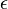# FAQ: How do we choose epsilon in the definition of stability?

(diff) ← Older revision | Latest revision (diff) | Newer revision → (diff)
If we can choosearbitrarily small, then stability of the equilibrium point is verified. It means that once I pick such, I can always find an initial condition that will guarantee that the trajectory x(t) (solution of the ODE describing my dynamical system of interest) will stay close to the equilibrium x_e, in a volume somehow delimited by the epsilon I chose (i.e. arbitrarily close to the equilibrium).
To verify if your equilibrium point is asymptotically stable, then according to the definition you are actually looking for anthat now delimits the distance between the initial condition and your equilibrium point. If as time increases the solution tends to the equilibrium, then lim_{t->inf}|| x-x_e|| =0 and the equilibrium is asymptotically stable.